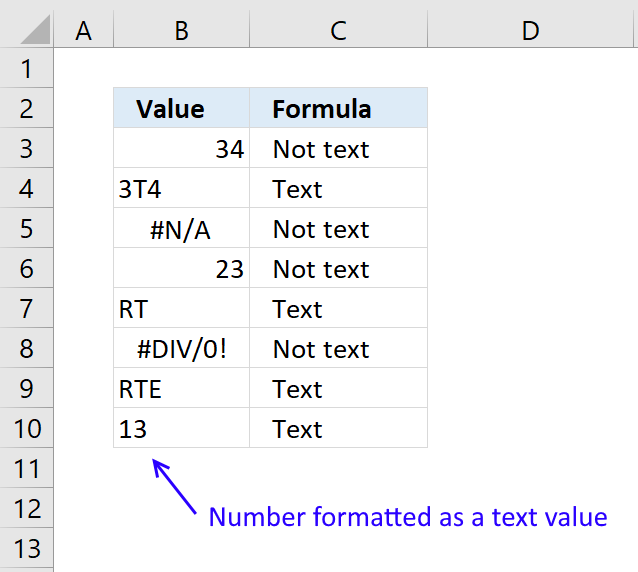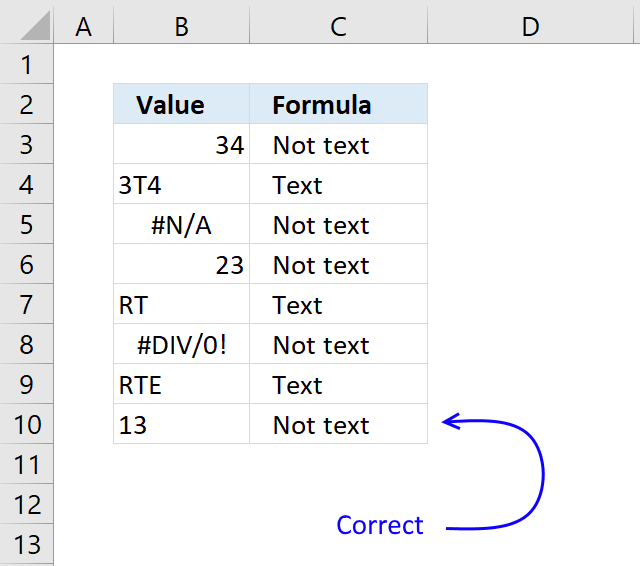Author: Oscar Cronquist Article last updated on April 11, 2018The picture above shows different values in column B and a formula in column C that tries to identifies the value in column B.

Formula in cell C3:

=IF(ISTEXT(B3),"Text","Not text")

The formula above checks if a cell contains a text value based on whether Excel correctly identified and formatted the cell as a text value or not.

This works often quite well, however, sometimes numbers are formatted as text.

This can happen if you import data from a database, copy and paste values from the web or a formula that returns numbers that Excel handles as a text string, among other things.

For example, cell B10 has a number formatted as a text value and the ISTEXT function incorrectly identifies the number as a text value.The following formula will correctly identify numbers even if Excel identifies the number as a text value.

Formula in cell C3:

=IF(ISTEXT(B3)*NOT(ISNUMBER(B3*1)),"Text","Not text")

### Explaining formula in cell C10

The formula in cell C10 is

=IF(ISTEXT(B10)*NOT(ISNUMBER(B10*1)),"Text","Not text")

B10*1 returns a number if B10 contains a number and an error for anything else.

13*1 = 13

ISNUMBER(B10*1) returns TRUE if the argument is a number and FALSE for all else.

ISNUMBER(B10*1) returns TRUE.

The NOT function returns TRUE if FALSE and FALSE if TRUE.

NOT(ISNUMBER(B10*1))

becomes

NOT(TRUE) and returns FALSE.

The ISTEXT function returns TRUE for all text values.

ISTEXT(B10) returns TRUE.

Multiplying the two functions is the same as AND logic. I could use the AND function, however, the * (asterisk) is smaller.

ISTEXT(B10)*NOT(ISNUMBER(B10*1))

becomes

TRUE*FALSE and returns 0. 1*0 = 0

The IF function then returns the third argument.

IF(ISTEXT(B10)*NOT(ISNUMBER(B10*1)),"Text","Not text")

becomes

IF(0,"Text","Not text")

and returns "Not text" in cell C10.